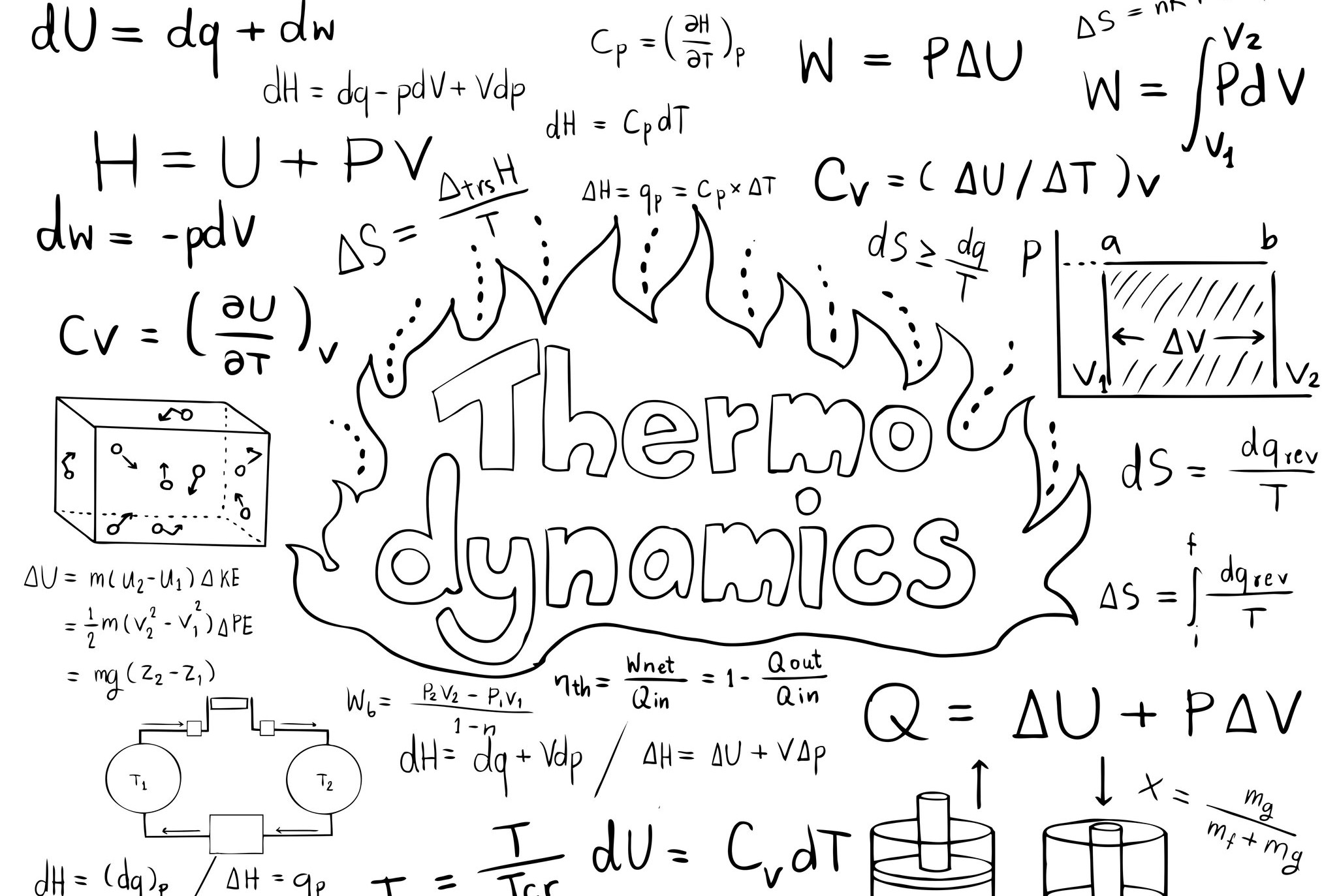# Energy balance of thermodynamics

When modeling thermal systems, there is no way around it: the energy balance, also known as the first law of thermodynamics. Most engineers are familiar with the energy balance from their studies, but we use an alternative formulation in the TIL Suite.Stefan Rauscher

|

December 22, 2021## Why an alternative formulation of the energy balance?

To model thermal systems, numerous other differential and algebraic equations are required besides the energy balance. For an efficient solution of the resulting differential-algebraic system of equations (DAE), a correct choice of the differential states is essential. The alternative formulation of the energybalance ensures that the specific enthalpy $$h$$ and the pressure $$p$$ are used as differential states when solving the DAE.

## It all begins with the control volume

Before the energy balance can be formulated, the control volume $$V$$ must be defined. Material flows and powers are transferred across the control volume’s boundaries. Within the control volume there is an ideally mixed fluid. To illustrate a control volume, a tank filled with water always beeing at a homogeneous temperature can be imagined.

All variables $$x$$ used in the following are understood as a function of time $$x = x(t)$$. For the sake of clarity, this dependence is not shown.

## Definition of the mass balance

The control volume’s mass balance can be written as:

$$\frac{dm}{dt} = \sum_i \dot{m}_i \tag{1}$$

The mass balance is very intuitive: the change in mass $$m$$ in the control volume over time $$t$$ is equal to the sum of all incoming and outgoing mass flow rates $$\dot{m}_i$$.

## Derivation of an alternative formulation of the energy balance

With the control volume and the mass balance defined, the alternative formulation of the energy balance used in the TIL Suite can be derived. The universal formulation of the energy balance is:

$$\frac{dE}{dt}= \sum_i \dot{m}_i (h_i + e_{a,i}) + \sum_j \dot{Q}_j + \sum_k P_k \tag{2}$$

The temporal change of the energy in the control volume $$\frac{dE}{dt}$$ results from the energies of the incoming and outgoing material flows $$\dot{m}_i(h_i +e_{a,i})$$, the heat flow rates $$\dot{Q}_j$$ and the mechanical powers $$P_k$$.

The total energy is defined as the sum of the internal energy $$U$$, the kinetic energy $$E_{kin}$$ and the potential energy $$E_{pot}$$, where the last two energies can be summarized as the external energy $$E_a$$:

$$E := U +E_{kin} + E_{pot} = U + E_a$$

A stationary system is considered. Thus, the temporal change of the external energies in the control volume $$\frac{dE_a}{dt}$$ can be neglected. Furthermore, the external energies of the material flows $$e_{a,i}$$ are neglected, since kinetic and potential energies only become relevant at large flow velocities and height differences. The term $$P_k$$ represents different forms of mechanical power. In the context of thermodynamic systems, typically only the power at volume change $$P_V = - p \frac{dV}{dt}$$ is of interest, where $$p$$ corresponds to the pressure in the control volume $$V$$. Overall, equation (2) can be simplified to:

$$\frac{dU}{dt}= \sum_i \dot{m}_i h_i + \sum_j \dot{Q}_j - p \frac{dV}{dt} \tag{3}$$

Using the relation $$U = H - pV$$, the internal energy can be expressed as a function of the enthalpy $$H$$ and the flow energy $$pV$$. By means of the product rule,the left side of equation (3) can be written as:

$$\frac{dU}{dt}= \frac{dH}{dt} - \frac{dpV}{dt} = \frac{dH}{dt} - V\frac{dp}{dt} -p\frac{dV}{dt}$$

Inserting this relation in equation (3) yields

$$\frac{dH}{dt}= \sum_i \dot{m}_i h_i + \sum_j \dot{Q}_j + V \frac{dp}{dt} \tag{4}$$

Note that equation (4) is still valid for variable control volumes. Next, the mass balance (1) is inserted into the left side of equation (4)

$$\frac{dH}{dt}= \frac{dmh}{dt} = m\frac{dh}{dt} + h\frac{dm}{dt} = m \frac{dh}{dt} + h \sum_i\dot{m}_i$$

where $$h$$ corresponds to the specific enthalpy in the control volume. Inserting this relation in equation (4) yields the alternative formulation of the energy balance:

$$m\frac{dh}{dt} = \sum_i \dot{m}_i (h_i - h) + \sum_j \dot{Q}_j + V\frac{dp}{dt}.$$M.Sc.

Stefan Rauscher

Simulation & Optimization

TLK Energy

Stefan Rauscher studied mechanical engineering at the RWTH Aachen University, specializing in energy engineering. He joined TLK Energy in 2018 and has since then been intensively involved in the simulation, control and optimization of thermal systems. He also regularly leads training on the simulation of thermal systems using the model library TIL.